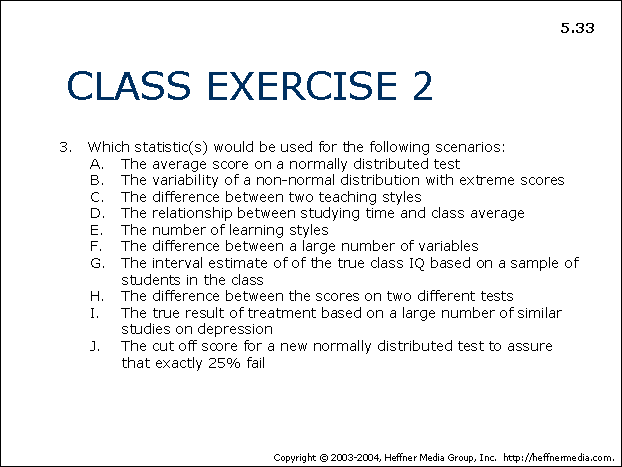# 33: Class Exercise 2: Using Statistics## CLASS EXERCISE 2

1. Which statistic(s) would be used for the following scenarios:
• A. The average score on a normally distributed test
• B. The variability of a non-normal distribution with extreme scores
• C. The difference between two teaching styles
• D. The relationship between studying time and class average
• E. The number of learning styles
• F. The difference between a large number of variables
• G. The interval estimate of the true class IQ based on a sample of students in the class
• H. The difference between the scores on two different tests
• I. The true result of treatment based on a large number of similar studies on depression
• J. The cut off score for a new normally distributed test to assure that exactly 25% fail